# Tag Archives: stem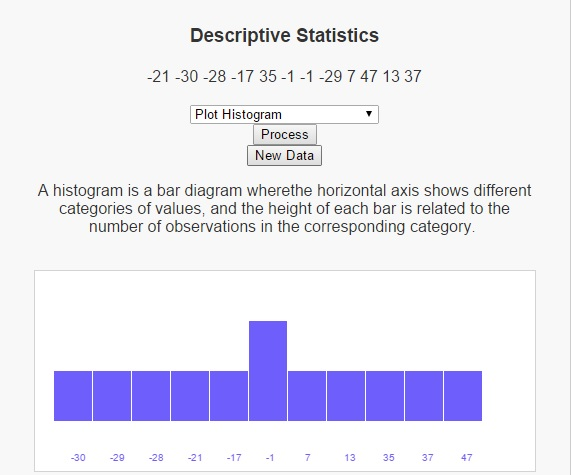I had been meaning to write a script and blog post on descriptive statistics for some time now, but with work and winter weather and the extra work that winter weather brings, and now that the winter weather is over trying to get back into an exercise routine (running up a hill is such a challenging experience, but when I get to the top of that hill I feel like Rocky Balboa on the steps at the steps at the entrance of the Philadelphia Museum of Art), I haven’t had the time to devote to this site that I would have liked. Well, that’s not entirely true. I have still been programming in my spare time. I just haven’t been able to share it here. I went to a conference in February and in my down time, I was able to write a script on descriptive statistics that I think gives a nice introduction to the area.

Before I go into descriptive statistics though, lets talk about statistics, which is concerned with the collection, analysis, interpretation and presentation of data. Statistics can generally be broken down into two categories, descriptive statistics and infernalinferential statistics, depending on what we would like to do with that data. When we are concerned with visualizing and summarizing the given data, descriptive statistics gives methods to operate on this data set. On the other hand, if we wish to draw conclusions about a larger population from our sample, then we would use methods from inferential statistics.

In the script on descriptive statistics I’ve written, I consider three different types of summaries for descriptive statistics:

Measures of Central Tendency
Mean – the arithmetic average of a set of values
Median – the middle number in a set of values
Mode – the most used number in a set of values

Dispersion
Maximum – the largest value in the data set
Minimum – the smallest value in the data set
Standard Deviation – the amount of variation in a set of data values
Variance – how far a set of numbers is spread out

Shape
Kurtosis – how peaked or flat a data set is
Skewness – how symmetric a data set is

Plots
Histogram Plots – a bar diagram where the horizontal axis shows different categories of values, and the height of each bar is related to the number of observations in the corresponding category.
Box and Whisker Plots – A box-and-whisker plot for a list of numbers consists of a rectangle whose left edge is at the first quartile of the data and whose right edge is at the third quartile, with a left whisker sticking out to the smallest value, and a right whisker sticking out to the largest value.
Stem and Leaf Plots – A stem and leaf plot illustrates the distribution of a group of numbers by arranging the numbers in categories based on the first digit.

# Polynomial ArithmeticWith students beginning to attend classes across the nation, I wanted to focus the site towards some of the things they’re going to be addressing. This latest page publicize some scripts that I wrote to help with polynomial arithmetic. Originally I wrote these as homework exercises for a class in programming, but I have found them useful ever since – both in teaching mathematics classes like college algebra, which spends a lot of attention on polynomials, and in my research life. Its funny (and sad) the number of simple errors that a person (mathematician or not) can make when performing simple arithmetic, so I found it very useful to have a calculator more advanced than the simple scientific calculators that are so easily available.

I’m not going to spend a lot of time discussing the importance of polynomials, or trying to justify their need. I will bring up some problems that I’d like to address in the future, that deal with polynomials. The first is finding the roots of the characteristic polynomial of a matrix. This is useful in research because these roots are the eigenvalues of the matrix and can give many properties of the matrix. There are also some data analysis tools like Singular Value Decomposition and Principal Component Analysis where I will probably build out from this initial set of instances.

The user interface for the scripts I’ve written generate two polynomials and ask the user what is to be done with those polynomials. The options are to add the two, subtract polynomial 2 from polynomial 1, multiply the two, divide polynomial 1 by polynomial 2, and divide polynomial 2 by polynomial 1. There is also the option to make the calculations more of a tutorial by showing the steps along the way. Users who want new problems can generate a new first or second polynomial and clear work.

For addition and subtraction, the program works by first ensuring that both polynomials have the same degree. This can be achieved by adding terms with zero coefficient to the lower degree polynomial. Once this has been accomplished, we simply add the terms that have the same exponent.

For multiplication, the program first builds a matrix A, where the element ai, i+j on row i and column i+j of the matrix A is achieved by multiplying the ith term of the first polynomial by the jth term of the second polynomial. If an was not given a value in the matrix, then we put a value of zero in that cell. Once this matrix is formed, we can sum the columns of the matrix to arrive at the final answer.

The division of two polynomials works first by dividing the first term of the numerator by the first term of the denominator. This answer is then multiplied by the denominator and subtracted from the numerator. Now, the first term in the numerator should cancel and we use the result as the numerator going froward. This process is repeated as long as the numerator’s degree is still equal to or greater than the denominator’s degree.

Check out the latest page on polynomial arithmetic and let me know what you think.

# Hidden Markov Models: The Baum-Welch Algorithm

Suppose you are at a table at a casino and notice that things don’t look quite right. Either the casino is extremely lucky, or things should have averaged out more than they have. You view this as a pattern recognition problem and would like to understand the number of ‘loaded’ dice that the casino is using and how these dice are loaded. To accomplish this you set up a number of Hidden Markov Models, where the loaded die are the latent (hidden) variables, and would like to determine which of these, if any is more likely to be using.

First lets go over a few things.

We will call each roll of the dice an observation. The observations will be stored in variables o1, o2, …, oT, where T is the number of total observations.

To generate a hidden Markov Model (HMM)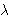we need to determine 5 parameters:

• The N states of the model, defined by S = {S1, …, SN}
• The M possible output symbols, defined by= {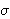1,2, …,M}
• The State transition probability distribution A = {aij}, where aij is the probability that the state at time t+1 is Sj, given that the state at time t is Si.
• The Observation symbol probability distribution B = {bj(k)} where bj(k) is the probability that the symbolk is emitted in state Sj.
• The initial state distribution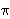= {i}, wherei is the probability that the model is in state Si at time t = 0.

The HMMs we’ve generated are based on two questions. For each question, you have provided 3 different answers which leads to 9 possible HMMs. Each of these models has its corresponding state transition and emission distributions.

• How often does the casino change dice?
• 0) Dealer Repeatedly Uses Same Dice
• 1) Dealer Uniformly Changes Die
• 2) Dealer Rarely Uses Same Dice
• Which sides on the loaded dice are more likely?
• 0) Larger Numbers Are More Likely
• 1) Numbers Are Randomly Likely
• 2) Smaller Numbers Are More Likely
How often does the casino change dice?
Which sides on
are more likely?
 (0, 0) (0, 1) (0, 2) (1, 0) (1, 1) (1, 2) (2, 0) (2, 1) (2, 2)

One of the interesting problems associated with Hidden Markov Models is called the Learning Problem, which asks the question “How can I improve a HMMso that it would be more likely to have generated the sequence O = o1, o2, …, oT?

The Baum-Welch algorithm answers this question using an Expectation-Maximization approach. It creates two auxiliary variables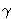t(i) and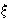t(i, j). The variablet(i) represents the probability of being in state i at time t, given the entire observation sequence. Likewiset(i, j) represents the joint probability of being in state i at time t and of being in state j at time t+1, given the entire observation sequence. They can be calculated byt(i) = (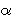t(i) *t(i) )j = 1 to N(t(j) *t(j))

andt(i, j) = (t(i) * ai, j *t+1(j) * bj(ot+1) )i’ = 1 to Nj’ = 1 to N(t(i’) * ai’, j’ *t+1(j’) * bj’(ot+1) )

As you can see, these are a direct result of calculations offrom the Forward algorithm andfrom the Backwards algorithm. Once we have calculated these variables, we can update the parameters of the model as follows: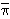i =1(i)i,j =t = 1 to T-1(t(i))t = t to T-1 (t(i, j))

// [b bar]_{j, k} = Sigma_{t = 1 to T, o_t = o_k} gamma_{t, j} / Sigma_{t = 1 to T} gamma_{t, j}, 1 <= j <= N, 1 <= k <= Mj(ok) =t = 1 to T-1, ot = okt(j)t = 1 to T-1t(j)

We can iterate this procedure a finite number of times or until it converges. This will generate a new model,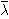= {N,,,,}.

There is more on this example at LEARNINGlover.com: Hidden Marokv Models: The Baum-Welch Algorithm.

Some further reading on Hidden Markov Models:

# The Risk of CompetitionI’m not a competitive person. Let me correct that. I try not to be a competitive person. I’ve recently been playing some of my favorite games from childhood like Monopoly, Chess, Spades, and Madden and I’ve been reminded of the competitive streak in me that hates losing. This streak has been relaxed in much of my adult life, and I tend to think that’s been to my benefit. Two pieces come to mind as I write this. One is a piece written by Slim Jackson of Single Black Male on the importance of asking questions. The second is what I heard on WAMU’s “Tell Me More” while driving home from work regarding the competitive nature generally assumed by (or dictated to) men.

These two concepts go hand in hand in my opinion because I’ve found that at times where I see a person as “my competition” I’m less likely to seek advice or help from that person. Doing this limits my resources and the set of people who can help me. I know that one of the hardest things to do while playing a game like Madden is asking my competition “how’d you just make that play” or listen to this competition explain how he knew to make such a play. However, its just this ability to swallow my pride and ask these questions that I’ve gotten better in Madden. I will admit that I’m not the most humble person on the face of the earth and so there are some things that I haven’t asked how to do. Generally in those things, I’ve found myself repeatedly playing the game trying to figure things out for myself.

There are applications of both sides of this to the real world. Some who love competition will say that it brings out the best in us, and they’d be sure to point out the feeling of satisfaction we get by working independently on a problem. As I look through my lists of inspirational quotes, I’m reminded of this with often repeated statements like “failure is the key to success”.

As rewarding as a competition can be, there’s also an important saying that we don’t need to re-invent the wheel. And what often gets lost in the do-it-yourself nature of competition is the ability to utilize all possible resources available to us. In particular, the skills of how to acknowledge the things we do not understand and how to formulate questions aimed at gaining understanding.

This brings us to is the other side of the competitive spirit. As rewarding as it is to be able to say, “wow, I can’t believe I was able to figure that out on my own”, it is also a stressful situation and there are many who are never able to say those words. Should these people be satisfied with failure?

I do not ask this in some devil’s advocate type of way. I ask coming from the point of view of a mathematician, an educator, and as a former student. I had a pretty dark moment in graduate school where I realized that my mere “love” of mathematics would not get me through qualifying exams. It wouldn’t suddenly make text books and academic papers instantly understandable. Suddenly I was placed in an uncomfortable position. Instead of always being the one who was the first to get the concept and who was leading the study groups on it, I’d be the one asking the questions. Looking at this in a “competitive” frame of mind (as I did then), I felt like I was losing the game.

This same moment though, is where my thinking was really changed. There was one thing, and one thing only that I would consider a failure and that was not finishing. I view everything else as a matter of swallowing my pride and readjusting my thought process to help get to that point.

Unfortunately though, many others do not get to this point. Many get lost in the scramble of the competition and do the equivalent of folding your hand in poker. They realize that at their current pace there is little to no chance that they’d win and so they just leave the game. And this is a real risk that we’re running with competition, particularly as STEM fields are becoming more and more important and we’re trying to encourage students to focus on these areas. What may be necessary to bring this about is a more cooperative approach to these things.